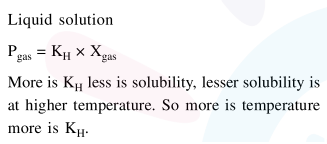# Which one of the following statements regarding Henry's law not correct ?

Question:

Which one of the following statements regarding Henry's law not correct ?

1. The value of $\mathrm{K}_{\mathrm{H}}$ increases with function of the nature of the gas

2. Higher the value of $\mathrm{K}_{\mathrm{H}}$ at a given pressure, higher is the solubility of the gas in the liquids.

3. The partial of the gas in vapour phase is proportional to the mole fraction of the gas in the solution.

4. Different gases have different $\mathrm{K}_{\mathrm{H}}$ (Henry's law constant) values at the same temnerature

Correct Option: , 2

Solution: